# Mesh Curvature node

Left Toolbar >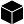Mesh Nodes >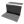The Mesh Curvature node computes the average curvature around each pixel of the 3D models and their normal images.

input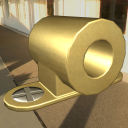Model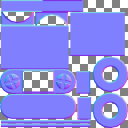Normal

output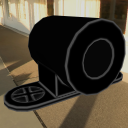CurvatureConvexConcaveMix

## Procedure

### To generate the Curvature image from a Mesh and its Normal image:

1. Load a Model into the Scene.

2. On the left toolbar choose Mesh Nodes > Mesh Curvature.>3. Drag the Mesh Curvature icon into the Node Graph.4. Connect a Normal image to the input socket in the Mesh Curvature node.

5. Connect the Output: Curvature, Convex or Concave.

A Normal Image.

## Outputs

Curvature
A monochromatic image where black represents flat areas and white represents curved areas.

Convex A monochromatic image where black represents flat areas and white represents convex areas.

Concave A monochromatic image where black represents flat areas and white represents concave areas.

Mix A monochromatic image where 50% gray represents flat areas, white represents convex areas, and black represents concave areas.

## Attributes PanelPosition X Position X of the output image.

Position Y Position Y of the output image.

Size X Size X of the output image.

Size Y Size Y of the output image.

Radius Defines a spherical area around each pixel that is measured for curvature.

Noise Adds contextual noise to the curvature output.

Normalize If checked gives the areas of greater curvature a white color. If unchecked, the color of the image is proportional to the surface curvature divided by the radius.

Combine If checked the curvature is computed including other elements in connected meshes in the object. If unchecked the curvature is computed only against the same element.

Padding Defines the size of the ring of pixels around the rendered zones, which prevents filtering issues when the model and textures are 3D rendered.